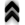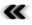## Appendix BRandom Number Generation

Anyone who attempts to generate random numbers by deterministic means is, of course, living in a state of sin.
Johann von Neumann

While it is mathematically suﬃcient to state a random number follows a speciﬁc probability density distribution function (Deﬁnition 100), this needs to be explicitly taken care of in a computing settings. The deterministic nature of digital computers is in stark contrast to any required randomness which therefore has to be introduced in an explicit manner. Specialized hardware devices which generate random numbers using various physical phenomena described by statistical means are among the available solutions to this issue. It is, however, also desirable to have a workable algorithmic solution. The reliance on a deterministic algorithm results in certain deﬁciencies of the generated numbers, as the algorithmic description is directly opposed to the concept of randomness. It thus also becomes necessary to consider the exact requirements the generated random numbers have to meet. This of course depends on the ﬁeld of application, such as Monte Carlo methods or cryptography. In the context of simulations, the eﬃcient, high performance generation of random numbers is as much a concern as the knowledge of the deﬁciencies. The exact reproducibility of a pseudo random sequence, which constitutes a mortal sin in the ﬁeld of cryptography , is not only perfectly acceptable in the context of Monte Carlo methods, where it may actually be considered a boon, as it eases the development and debugging of algorithms.

The pseudo random sequences generated in an algorithmic fashion experience a period after which the sequence repeats itself. Applications utilizing any such sequence need to be aware of this limitation and should accomplish their calculations before the period is exhausted .

Furthermore, care has to be taken, if multiple pseudo random generators are used in order to avoid erroneous results due to unchecked correlations in the generated sequences. This issue is of increased signiﬁcance due to the general trend towards parallelization .

The generation of sequences of pseudo random numbers depends on the desired probability density distribution function. Since it is impractical to provide a pseudo random generator for any conceivable probability distribution function, a possibility to derive pseudo random sequences with diﬀering probability density functions from one another is highly desirable.

One way to accomplish this is using the basic expression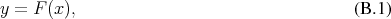ﬁnding an inverse function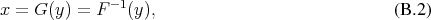and using it to calculate the desired probability distribution. This method, however, obviously requires that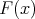to be invertible, which is not only a theoretical restriction, but may also pose computational challenges.

As inversion may not be a viable course of action, diﬀerent procedures have been developed such as the rejection technique, which is also called the rejection sampling method or Neumann’s method. Using an input pseudo random variable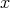of an available distribution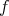, it calculates a pseudo random number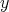of an arbitrary output distribution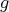. For this task it requires a rejection and acceptance rule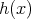, which determines, if a pseudo random number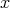is viable or not. In case the rule is met, the random number with the desired distribution is calculated using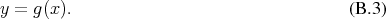The eﬃciency of such a procedure depends on a ﬁtting choice of the acceptance rule.

When the desired probability distribution function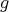is representable in the form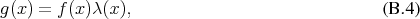where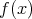is the input probability distribution density function connected to a variable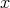and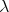a bounded function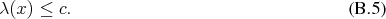With these settings the algorithm takes the form:
1.
Generate a pseudo random variable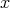according to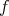.
2.
Generate a pseudo random variable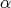– usually the distribution is chosen to be uniform .
3.
If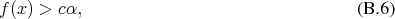accept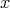as a realization of a random variable distributed according to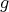. Otherwise, reject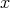and restart the procedure.